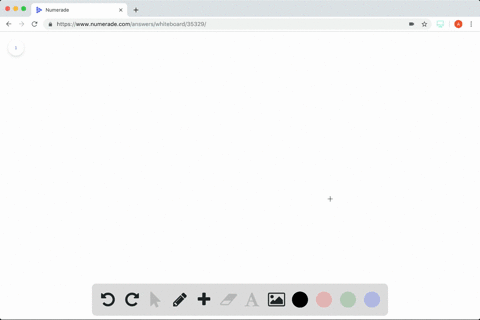Enroll in one of our FREE online STEM summer camps. Space is limited so join now!View Summer Courses### Finding a Particular Solution In Exercises $37-44… 04:09University of Houston Need more help? Fill out this quick form to get professional live tutoring. Get live tutoring Problem 41 Finding a Particular Solution In Exercises$37-44,$find the particular solution of the differential equation that satisfies the initial condition(s).$f^{\prime \prime}(x)=2, f^{\prime}(2)=5, f(2)=10\$

$$f(x)=x^{2}+x+4$$

## Discussion

You must be signed in to discuss.

## Video Transcript

theory in 41 they wanted to find a particular solution, but we haven't given up. So the facts that were given is double prime. It was too. And yeah, Rhine and Exit, too. Why? Five? And in a position to All right. So down. Thank you. In degree. Her so fine. It would be cool beings, chieftains to x see. Never gonna solve for C So a crime would be by from here equals two times team won't see So I buy those board can see c one my equation for a crime. It x is going to be equal to X. That's one. You take the grow Well Mmm. Thanks. He won't you know, It's plus one. The s Thank you. Q x as my ex cuse me spare multiplied by Yeah, yeah, No. See? So when I re write this 1/2 times two is just one you have of eggs equals X where see? And I have to find out what sees. So I'm point substitute 10 in for Dex and to the next there is for us to see bean Thanks. And before more sea equals four. So my equation for f X comes Thanks. We're thanks. Yes,